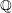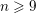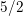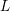Home
Hostname: page-component-568f69f84b-56sbs Total loading time: 0.155 Render date: 2021-09-23T07:07:29.672Z Has data issue: true Feature Flags: { "shouldUseShareProductTool": true, "shouldUseHypothesis": true, "isUnsiloEnabled": true, "metricsAbstractViews": false, "figures": true, "newCiteModal": false, "newCitedByModal": true, "newEcommerce": true, "newUsageEvents": true }Compositio Mathematica

# Rational points on hyperelliptic curves having a marked non-Weierstrass point

Published online by Cambridge University Press:  09 October 2017

## Abstract

In this paper, we consider the family of hyperelliptic curves over$\mathbb{Q}$ having a fixed genus$n$ and a marked rational non-Weierstrass point. We show that when$n\geqslant 9$ , a positive proportion of these curves have exactly two rational points, and that this proportion tends to one as$n$ tends to infinity. We study rational points on these curves by first obtaining results on the 2-Selmer groups of their Jacobians. In this direction, we prove that the average size of the 2-Selmer groups of the Jacobians of curves in our family is bounded above by 6, which implies a bound of$5/2$ on the average rank of these Jacobians. Our results are natural extensions of Poonen and Stoll [Most odd degree hyperelliptic curves have only one rational point, Ann. of Math. (2) 180 (2014), 1137–1166] and Bhargava and Gross [The average size of the 2-Selmer group of Jacobians of hyperelliptic curves having a rational Weierstrass point, in Automorphic representations and$L$ -functions, Tata Inst. Fundam. Res. Stud. Math., vol. 22 (Tata Institute of Fundamental Research, Mumbai, 2013), 23–91], where the analogous results are proved for the family of hyperelliptic curves with a marked rational Weierstrass point.

## MSC classification

Type
Research Article
Information
Compositio Mathematica , January 2018 , pp. 188 - 222

## Access options

Get access to the full version of this content by using one of the access options below. (Log in options will check for institutional or personal access. Content may require purchase if you do not have access.)

## References

Bektemirov, B., Mazur, B., Stein, W. and Watkins, M., Average ranks of elliptic curves: tension between data and conjecture , Bull. Amer. Math. Soc. (N.S.) 44 (2007), 233254 (electronic).CrossRefGoogle Scholar
Bhargava, M., The density of discriminants of quartic rings and fields , Ann. of Math. (2) 162 (2005), 10311063.CrossRefGoogle Scholar
Bhargava, M., The density of discriminants of quintic rings and fields , Ann. of Math. (2) 172 (2010), 15591591.CrossRefGoogle Scholar
Bhargava, M., Most hyperelliptic over$\mathbb{Q}$ curves have no rational points, Preprint (2013), arXiv:1308.0395.Google Scholar
Bhargava, M. and Gross, B., The average size of the 2-Selmer group of Jacobians of hyperelliptic curves having a rational Weierstrass point , in Automorphic representations and L-functions, Tata Inst. Fundam. Res. Stud. Math., vol. 22 (Tata Institute of Fundamental Research, Mumbai, 2013), 2391.Google Scholar
Bhargava, M. and Gross, B., Arithmetic invariant theory , in Symmetry: representation theory and its applications, Progress in Mathematics, vol. 257 (Birkhäuser, New York, 2014), 3354.Google Scholar
Bhargava, M. and Shankar, A., Binary quartic forms having bounded invariants, and the boundedness of the average rank of elliptic curves , Ann. of Math. (2) 181 (2015), 191242.CrossRefGoogle Scholar
Bhargava, M., Shankar, A. and Wang, X., Squarefree values of polynomial discriminants I, Preprint (2016), arXiv:1611.09806.Google Scholar
Borel, A., Ensembles fondamentaux pour les groupes arithmétiques , in Colloque sur la Théorie des Groupes Algébriques, Bruxelles (1962), 2340.Google Scholar
Bosch, S., Lutkebohmert, W. and Raynaud, M., Néron models, Ergebnisse der Mathematik und ihrer Grenzgebiete (3), vol. 21 (Springer, Berlin, 1990).CrossRefGoogle Scholar
Chabauty, C., Sur les points rationnels des courbes algébriques de genre supérieur à l’unité , C. R. Acad. Sci. Paris 212 (1941), 882885.Google Scholar
Coleman, R., Effective Chabauty , Duke Math. J. 52 (1985), 765770.CrossRefGoogle Scholar
Davenport, H., On a principle of Lipschitz , J. Lond. Math. Soc. 26 (1951), 179183. Corrigendum: ‘On a principle of Lipschitz’, J. Lond. Math. Soc. 39 (1964), 580.CrossRefGoogle Scholar
Denef, J. and van den Dries, L., p-adic and real subanalytic sets , Ann. of Math. (2) 128 (1988), 79138.CrossRefGoogle Scholar
Langlands, R. P., The volume of the fundamental domain for some arithmetical subgroups of Chevalley groups , in Algebraic groups and discontinuous subgroups, Proceedings of Symposia in Pure Mathematics, vol. 9 (American Mathematical Society, Providence, RI, 1966), 143148.CrossRefGoogle Scholar
Platonov, V. and Rapinchuk, A., Algebraic groups and number theory, Pure and Applied Mathematics, vol. 139 (Academic Press, Boston, MA, 1994). Translated from the 1991 Russian original by Rachel Rowen.Google Scholar
Poonen, B. and Schaefer, E., Explicit descent for Jacobians of cyclic covers of the projective line , J. Reine Angew. Math. 488 (1997), 141188.Google Scholar
Poonen, B. and Stoll, M., Most odd degree hyperelliptic curves have only one rational point , Ann. of Math. (2) 180 (2014), 11371166.CrossRefGoogle Scholar
Serre, J. P., A course in arithmetic, Graduate Texts in Mathematics, vol. 7 (Springer, New York, 1973).CrossRefGoogle Scholar
Stoll, M., Implementing 2-descent for Jacobians of hyperelliptic curves , Acta Arithmetica XCVIII.3 (2001), 245277.CrossRefGoogle Scholar
Wang, X., Maximal linear spaces contained in the base loci of pencils of quadrics, Preprint (2013), arXiv:1302.2385.Google Scholar
Wang, X., Pencils of quadrics and Jacobians of hyperelliptic curves, PhD thesis, Harvard University (2013).Google Scholar
1
Cited by

# Send article to Kindle

Note you can select to send to either the @free.kindle.com or @kindle.com variations. ‘@free.kindle.com’ emails are free but can only be sent to your device when it is connected to wi-fi. ‘@kindle.com’ emails can be delivered even when you are not connected to wi-fi, but note that service fees apply.

Find out more about the Kindle Personal Document Service.

Rational points on hyperelliptic curves having a marked non-Weierstrass point
Available formats
×

# Send article to Dropbox

To send this article to your Dropbox account, please select one or more formats and confirm that you agree to abide by our usage policies. If this is the first time you use this feature, you will be asked to authorise Cambridge Core to connect with your <service> account. Find out more about sending content to Dropbox.

Rational points on hyperelliptic curves having a marked non-Weierstrass point
Available formats
×

# Send article to Google Drive

To send this article to your Google Drive account, please select one or more formats and confirm that you agree to abide by our usage policies. If this is the first time you use this feature, you will be asked to authorise Cambridge Core to connect with your <service> account. Find out more about sending content to Google Drive.

Rational points on hyperelliptic curves having a marked non-Weierstrass point
Available formats
×
×